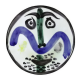# Phrases Starting With Numbers | part 4

Last chance…How I See It

This is the last set of phrases. The previous parts are here, here, and here.

Each entry is a phrase that begins with a number. For example: 4 = S on a S could be 4 Sides on a Square. Relax your mind. Put some replies into the comment box.

14 = D in a F
206 = B in the HB
52 = W in a Y
24 = BBB in a P
76 = T led the BP
12 = D of C
3 = C in the F
6 = P on the S of D
77 = SS
4 = B’s (J,P,R, & G)
31 = BRF
16 = and NBK
18 = and YCV
18 = W on a TTT
1 = GTDA
60 = M in an H
10 = LIB

View original post

This entry was posted in Game and tagged , , , , on by .